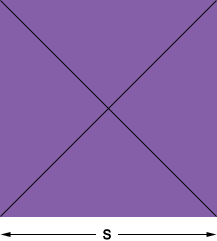SEARCH HOMEMath Central Quandaries & QueriesQuestion from Kimi, a student: A quilt square is stitched along each diagonal to make 4 right triangles. Each diagonal is 12 inches long. ( 1 foot) How many quilt squares can be cut from a piece of fabric that is 8 feet long and 2 feet wide??Hi Kimi,

I would first convert all the measurements to inches.

Here is my quilt square, it has a side length of s inches.Look at the bottom right triangle. It has two sides of length 6 inches each and a hypotenuse of length s inches. Use Pythagoras theorem to find s. How many squares of side length s inches can you cut from your piece of fabric?

PennyMath Central is supported by the University of Regina and The Pacific Institute for the Mathematical Sciences.